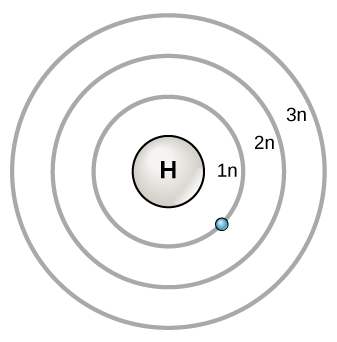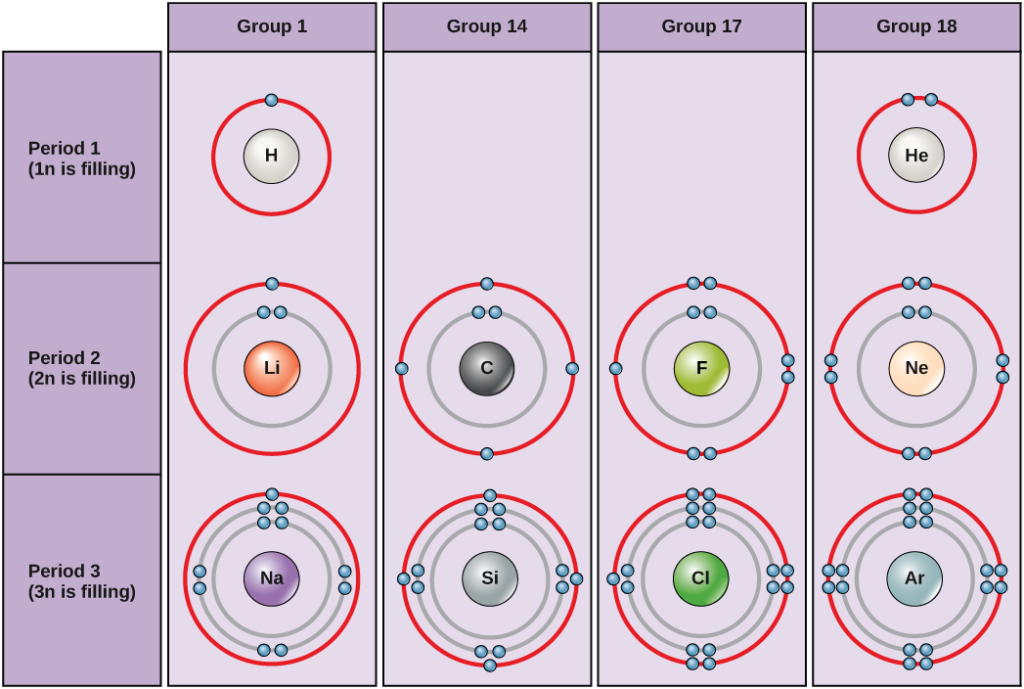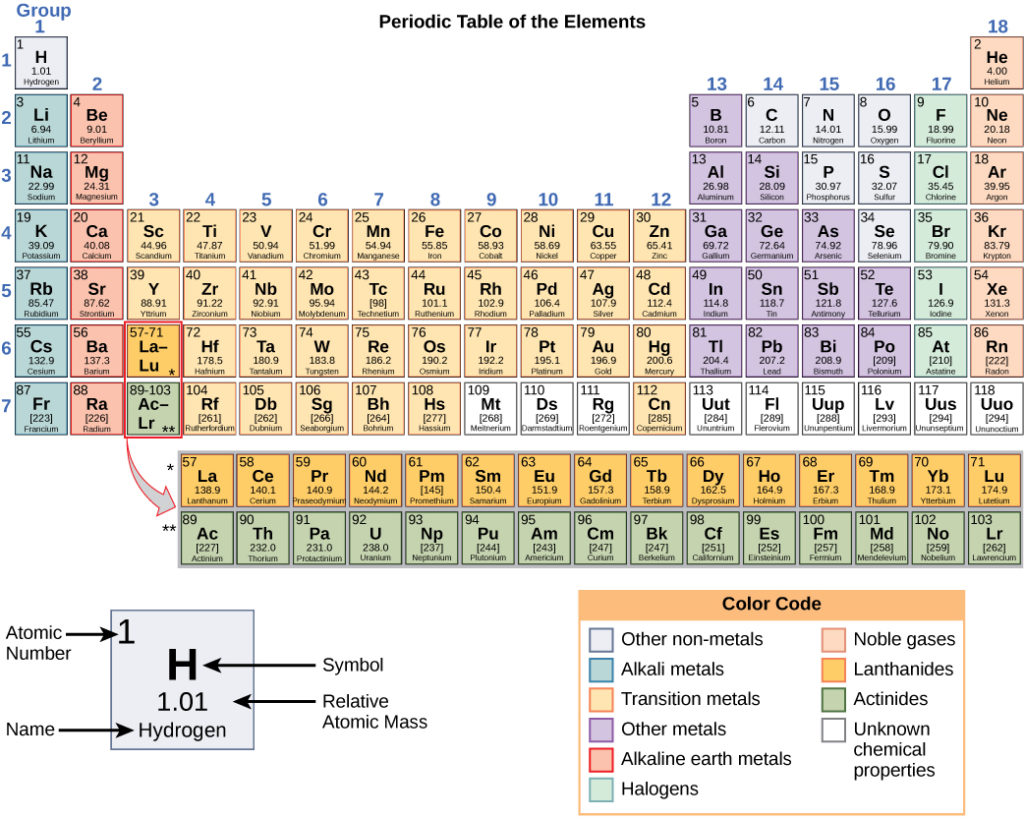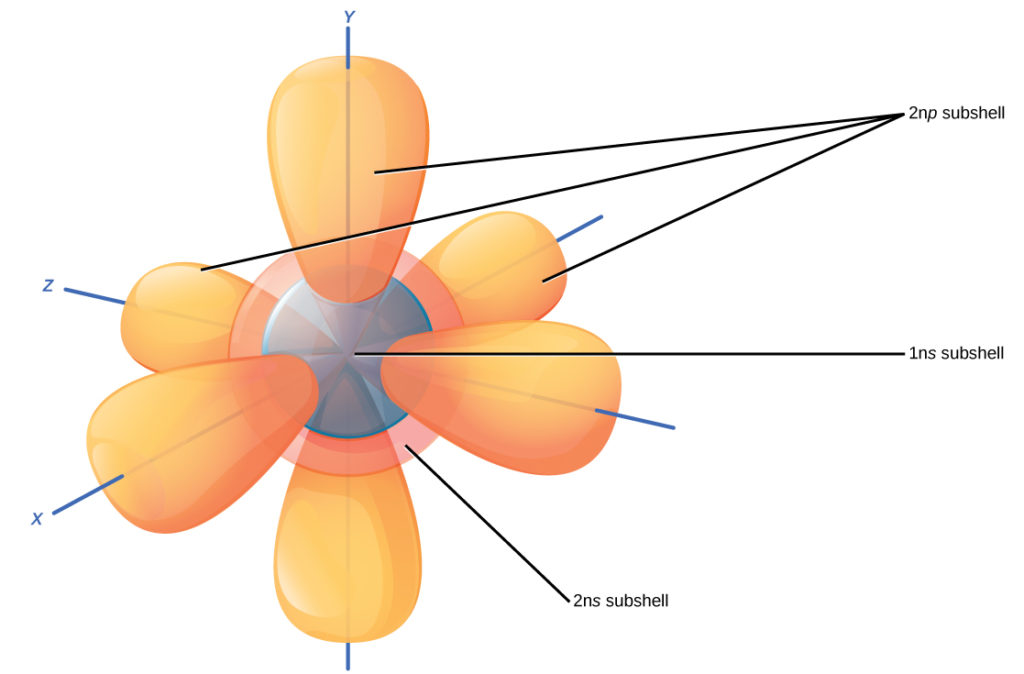## Electrons

### Learning Outcomes

• Describe the behavior and location of electrons, and how these factors influence bond formation between atoms

## Electron Shells and the Bohr ModelFigure 1. The Bohr model.

It should be stressed that there is a connection between the number of protons in an element, the atomic number that distinguishes one element from another, and the number of electrons it has. In all electrically neutral atoms, the number of electrons is the same as the number of protons. Thus, each element, at least when electrically neutral, has a characteristic number of electrons equal to its atomic number.

An early model of the atom was developed in 1913 by Danish scientist Niels Bohr (1885–1962). In this model, electrons exist within principal shells. An electron normally exists in the lowest energy shell available, which is the one closest to the nucleus. Energy from a photon of light can bump it up to a higher energy shell, but this situation is unstable, and the electron quickly decays back to the ground state. In the process, a photon of light is released.

The Bohr model shows the atom as a central nucleus containing protons and neutrons, with the electrons in circular orbitals at specific distances from the nucleus, as illustrated in Figure 1. These orbits form electron shells or energy levels, which are a way of visualizing the number of electrons in the outermost shells. These energy levels are designated by a number and the symbol “n.” For example, 1n represents the first energy level located closest to the nucleus.

Electrons fill orbitals in a consistent order: they first fill the orbitals closest to the nucleus, then they continue to fill orbitals of increasing energy further from the nucleus. If there are multiple orbitals of equal energy, they will be filled with one electron in each energy level before a second electron is added. The electrons of the outermost energy level determine the energetic stability of the atom and its tendency to form chemical bonds with other atoms to form molecules.

Under standard conditions, atoms fill the inner shells first, often resulting in a variable number of electrons in the outermost shell. The innermost shell has a maximum of two electrons but the next two electron shells can each have a maximum of eight electrons. This is known as the octet rule, which states, with the exception of the innermost shell, that atoms are more stable energetically when they have eight electrons in their valence shell, the outermost electron shell. Examples of some neutral atoms and their electron configurations are shown in Figure 2. Notice that in this figure, helium has a complete outer electron shell, with two electrons filling its first and only shell. Similarly, neon has a complete outer 2n shell containing eight electrons. In contrast, chlorine and sodium have seven and one in their outer shells, respectively, but theoretically they would be more energetically stable if they followed the octet rule and had eight.

### Practice QuestionFigure 2. Bohr diagrams for hydrogen, helium, lithium, carbon, fluorine, neon, sodium, silicon, chlorine, and argon.

Bohr diagrams indicate how many electrons fill each principal shell. Group 18 elements (helium, neon, and argon are shown in Figure 2) have a full outer, or valence, shell. A full valence shell is the most stable electron configuration. Elements in other groups have partially filled valence shells and gain or lose electrons to achieve a stable electron configuration.

An atom may give, take, or share electrons with another atom to achieve a full valence shell, the most stable electron configuration. Looking at this figure, how many electrons do elements in group 1 need to lose in order to achieve a stable electron configuration? How many electrons do elements in groups 14 and 17 need to gain to achieve a stable configuration?

Understanding that the organization of the periodic table is based on the total number of protons (and electrons) helps us know how electrons are distributed among the outer shell. The periodic table is arranged in columns and rows based on the number of electrons and where these electrons are located. Take a closer look at the some of the elements in the periodic table’s far right column in Figure 3.Figure 3. The periodic table shows the atomic mass and atomic number of each element. The atomic number appears above the symbol for the element and the approximate atomic mass appears below it.

The group 18 atoms helium (He), neon (Ne), and argon (Ar) all have filled outer electron shells, making it unnecessary for them to share electrons with other atoms to attain stability; they are highly stable as single atoms. Their non-reactivity has resulted in their being named the inert gases (or noble gases). Compare this to the group 1 elements in the left-hand column. These elements, including hydrogen (H), lithium (Li), and sodium (Na), all have one electron in their outermost shells. That means that they can achieve a stable configuration and a filled outer shell by donating or sharing one electron with another atom or a molecule such as water. Hydrogen will donate or share its electron to achieve this configuration, while lithium and sodium will donate their electron to become stable. As a result of losing a negatively charged electron, they become positively charged ions. Group 17 elements, including fluorine and chlorine, have seven electrons in their outmost shells, so they tend to fill this shell with an electron from other atoms or molecules, making them negatively charged ions. Group 14 elements, of which carbon is the most important to living systems, have four electrons in their outer shell allowing them to make several covalent bonds (discussed below) with other atoms. Thus, the columns of the periodic table represent the potential shared state of these elements’ outer electron shells that is responsible for their similar chemical characteristics.

## Electron Orbitals

Although useful to explain the reactivity and chemical bonding of certain elements, the Bohr model of the atom does not accurately reflect how electrons are spatially distributed surrounding the nucleus. They do not circle the nucleus like the earth orbits the sun, but are found in electron orbitals. These relatively complex shapes result from the fact that electrons behave not just like particles, but also like waves. Mathematical equations from quantum mechanics known as wave functions can predict within a certain level of probability where an electron might be at any given time. The area where an electron is most likely to be found is called its orbital.Figure 4. Click for a larger image. The s subshells are shaped like spheres. Both the 1n and 2n principal shells have an s orbital, but the size of the sphere is larger in the 2n orbital. Each sphere is a single orbital. p subshells are made up of three dumbbell-shaped orbitals. Principal shell 2n has a p subshell, but shell 1 does not.

Recall that the Bohr model depicts an atom’s electron shell configuration. Within each electron shell are subshells, and each subshell has a specified number of orbitals containing electrons. While it is impossible to calculate exactly where an electron is located, scientists know that it is most probably located within its orbital path. Subshells are designated by the letters sp, d, and f. The subshell is spherical in shape and has one orbital. Principal shell 1n has only a single s orbital, which can hold two electrons. Principal shell 2n has one s and one p subshell, and can hold a total of eight electrons. The p subshell has three dumbbell-shaped orbitals, as illustrated in Figure 4. Subshells d and f have more complex shapes and contain five and seven orbitals, respectively. These are not shown in the illustration. Principal shell 3n has s, p, and d subshells and can hold 18 electrons. Principal shell 4n has s, p, d and orbitals and can hold 32 electrons. Moving away from the nucleus, the number of electrons and orbitals found in the energy levels increases. Progressing from one atom to the next in the periodic table, the electron structure can be worked out by fitting an extra electron into the next available orbital.

The closest orbital to the nucleus, called the 1s orbital, can hold up to two electrons. This orbital is equivalent to the innermost electron shell of the Bohr model of the atom. It is called the 1s orbital because it is spherical around the nucleus. The 1s orbital is the closest orbital to the nucleus, and it is always filled first, before any other orbital can be filled. Hydrogen has one electron; therefore, it has only one spot within the 1s orbital occupied. This is designated as 1s1, where the superscripted 1 refers to the one electron within the 1s orbital. Helium has two electrons; therefore, it can completely fill the 1s orbital with its two electrons. This is designated as 1s2, referring to the two electrons of helium in the 1s orbital. On the periodic table Figure 4, hydrogen and helium are the only two elements in the first row (period); this is because they only have electrons in their first shell, the 1s orbital. Hydrogen and helium are the only two elements that have the 1s and no other electron orbitals in the electrically neutral state.

The second electron shell may contain eight electrons. This shell contains another spherical s orbital and three “dumbbell” shaped p orbitals, each of which can hold two electrons, as shown in Figure 4. After the 1s orbital is filled, the second electron shell is filled, first filling its 2s orbital and then its three p orbitals. When filling the p orbitals, each takes a single electron; once each p orbital has an electron, a second may be added. Lithium (Li) contains three electrons that occupy the first and second shells. Two electrons fill the 1s orbital, and the third electron then fills the 2s orbital. Its electron configuration is 1s22s1. Neon (Ne), on the other hand, has a total of ten electrons: two are in its innermost 1s orbital and eight fill its second shell (two each in the 2s and three p orbitals); thus, it is an inert gas and energetically stable as a single atom that will rarely form a chemical bond with other atoms. Larger elements have additional orbitals, making up the third electron shell. While the concepts of electron shells and orbitals are closely related, orbitals provide a more accurate depiction of the electron configuration of an atom because the orbital model specifies the different shapes and special orientations of all the places that electrons may occupy.

Watch this visual animation to see the spatial arrangement of the p and s orbitals. Note that this video has no audio.

### Video Review

This video gives another overview of the electron:

## Contribute!

Did you have an idea for improving this content? We’d love your input.